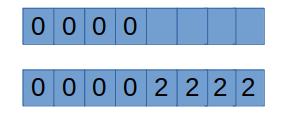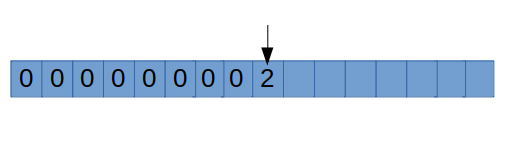5G时代下一个聚合的编程学习网

# [算法导论]#1 摊还分析

## 引言

• 建立一个表，初始化大小为1．
• 如果表的容量不够，那么就把大小扩大为原来的两倍，将原来表的内容复制一遍
• 把原来表的内存释放，再执行插入
• 容量会以1,2,4,8,16……(2的幂次)的增长

• 当表里存在(n)个元素，最坏复杂度是(O(n)​)
• (n)个元素插入的最坏复杂度是(O(n^2)​)

## 聚集分析

[n+sum_{j=0}^{lfloor lg(n-1)floor} 2^j
]

[sum_{j=0}^{lfloor log_2(n-1)floor} 2^j<=2n
]

• 平摊分析可以用来证明在一系列操作中，通过对所有操作求平均之后，即使其中单一的操作具有较大的代价，平均代价还是很小的
• 平摊分析与平均情况分析的不同之处在于它不牵涉到概率
• 平摊分析保证在最坏情况下，每个操作具有平均性能。

## 记账方法

[sum_{i=1}^{n}C_ileq sum_{i=1}^{n}check C_i
]

• 初始设置(check C_i=3​)，其中1元用于插入，2元用于将表翻倍的预存费用．

• 当表扩大两倍，就从存款里取出1美元来移动新项，还有1美元来移动旧项．

• 当长度为8的时候:

里面的数字就是余额，前四个余额为0，这时开始执行插入操作

花费1元用于插入，余额为2元，插入四个之后：• 这时动态表需要扩大两倍，扩大两倍需要多少钱?没错，非常完美地，余额为8刚好可以支付复制操作，然后新插入的第九项也是花费1元进行插入，余额为2元．## 势能法

• 操作(i)(D_{i-1})转化为(D_i)，操作(i)的操作代价还是\$ C_i \$

• 定义势能函数(phi)，将数据结构映射为实数

• 这样初始的势能是(phi (D_0)=0) ，对于所有(i)都有\$phi (D_0) geq 0 \$

• 平摊代价(check C_i=C_i+phi(D_i)-phi(D_{i-1}))

• (Delta phi_i=phi(D_i)-phi(D_{i-1})​)是势能的改变量

• 如果(Delta phi_i geq 0) ，第(i)次操作存储了后面数据结构所需的代价
• 如果(Delta phi _i leq0)， 第(i)次操作消耗了之前数据结果存储的代价

[sum _{i=1}^{n}check C_i = sum_{i=1}^{n}(C_i+phi(D_i)-phi(D_{i-1}))
]

[=sum_{i=1}^{n}C_i+phi(D_n)-phi(D_0)　　　 phi(D_0)=0 　　phi(D_n) geq 0
]

[geq sum_{i=1}^{n}C_i
]

• 定义势能函数(phi(D_i)=2i-2^{lceil logi ceil})

• 可得(phi(D_0)=2^{log0}=0)

• 因为(lceil lgi ceil​)向上取整，所以最大取到\$ lgi +1​\$，所以(2^{lceil lgi ceil}​)最大取到(2i​)(phi(D_i)ge0​)

• 由\$ check C_i=C_i+phi(D_i)-phi(D_{i-1})\$

• 实际代价(C_i)由上文可知分两种情况，一般情况下是(1)，而当(i-1)(2)的幂时为(i)

[phi(D_i)-phi(D_{i-1})＝(2i-2^{lceil lgi ceil})-(2(i-1)-2^{lceil lg(i-1)ceil})
]

[=2-2^{lceil lgi ceil}+2^{lceil lg(i-1) ceil}
]

• 分情况分析:当(i-1)(2)的幂次时:

[check C_i=i+2-2^{lceil lgi ceil}+2^{lceil lg(i-1) ceil}
]

[= i+2-2(i-1)+i-1
=3
]

• 其他情况:

[check C_i=1+2-2^{lceil lgi ceil}+2^{lceil lg(i-1) ceil}
]

[=1+2=3
]

## 总结

• 平摊分析为数据结构的性能提供了一个简洁的抽象概念。
• 在不关注实时表现，只关注聚集行为时，平摊分析是一种很好的描述方式。
• 在有些情况中，不同的操作会有不同的平摊代价，它们的和仍然是实际代价的上限。
• 三种方法都是可用的，但都有各自的适用情况使得它们是简单准确的，不同的方法可能得到不同的上限结果。